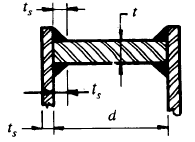Related Resources: pressure-vessel

### Flat Circular Head Welded with Internal or External Pressure Equation and Calculator

Circular Flat Head Welded with Internal or External Pressure Equation and CalculatorALL calculators require a Premium Membership

Preview

Required minimum wall thickness
t = d ( C P / ( S E) )0.5

C = 0.33 tr / ts

Cmin = 0.20

Where:

P = Design pressure or max. allowable working pressure, psi
d = Inside diameter of vessel , inches
S = Rated stress value of the material
t = Minimum thickness of shell (wall) exclusive of corrosion, inches
th = Actual thickness used in actual design, inches
tr = Minimum required thickness of seamless shell for pressure, inches
ts = Actual thickness of shell, exclusive of corrosion allowance, inches
E = Weld Joint efficiency

Example Design Calculations:

P = 300 psi design pressure
E= joint efficiency
d = 24in. inside diameter of shell
s =15,000 psi maximum allowable stress value of plate
tr = 0.243 in. required thickness of seamless shell for pressure.
ts = 0.3125 in. actual thickness of shell.

C = 0.330 ( 0.234 in. / 0.3125 in. ) = 0.260

t = 24 in ( 0.26 in x 300 psi / ( 15,000 psi x 1.0 ) = 1.731 in.

Design use 1.75 in. plate

If using a thicker plate for shell, a less thick plate would be satisfactory for the head.

ts = 0.375 in. actual thickness of shell.

C = 0.330 ( 0.234 in. / 0.375 in. ) = 0.214

t = 24 in ( 0.214 in x 300 psi / ( 15,000 psi x 1.0 ) = 1.57 in.

Design use 1.625 in. plate

The shell thickness should be maintained along a distance 2 ( d ts )0.5 from the inside face of the head

2 ( 24in. 0.375 in.)0.5 = 6 in.